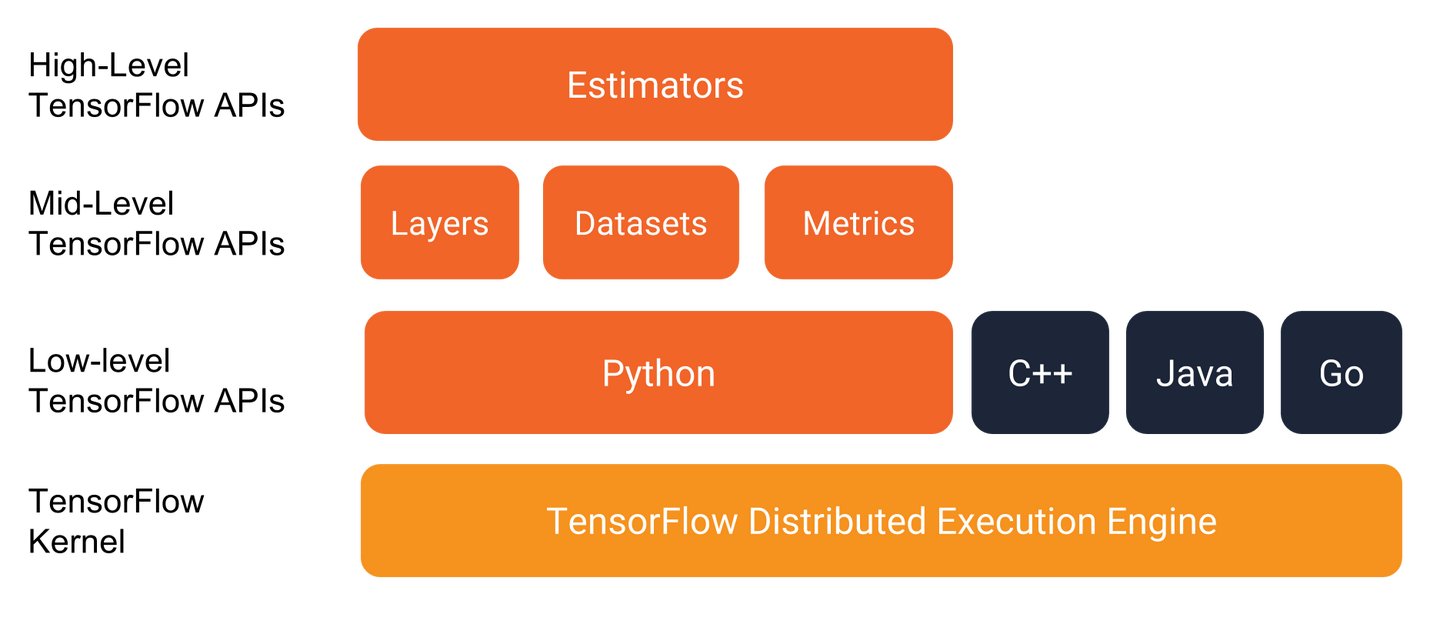## 目录

• Dataset API
• TFRecord

• 数据说明
• 数据存储
• 常用存储
• TFRecord存储

• 生成数据
• 写入TFRecord file
• 存储类型
• 如何存储张量feature
• 使用Dataset
• 创建dataset
• 操作dataset
• 解析函数
• 迭代样本
• Shuffle
• Batch
• Epoch

## 前言

YJango/TFRecord-Dataset-API

## 优势

1. 简洁性：

• Dataset API：将数据直接放在graph中进行处理，整体对数据集进行上述数据操作，使代码更加简洁。

2. 对接性：TensorFlow中也加入了高级API （Estimator、Experiment，Dataset）帮助建立网络，和Keras等库不一样的是：这些API并不注重网络结构的搭建，而是将不同类型的操作分开，帮助周边操作。可以在保证网络结构控制权的基础上，节省工作量。若使用Dataset API导入数据，后续还可选择与Estimator对接。

## 概念

1. 训练：在每一步训练中，神经网络会把输入和 正确的输出送入中来更新一次神经网络中的参数。用很多个不同的不断更新，最终希望当遇到新的时，可以用判断出正确的2. 专有名词：结合下图说明名称

• 样本 (example)：：输入和 正确的输出一起叫做样本。给网络展示了什么输入该产生什么样的输出。这里每个是五维向量，每个是一维向量。
• 表征 (representation)：：集合了代表个人的全部特征。
• 特征 (feature)：中的某个维：如年龄，职业。是某人的一个特点。
• 标签 (label)：：正确的输出。

1. 常用存储：

2. TFRecord存储：

TFRecord是以字典的方式一次写一个样本，字典的keys可以不以输入和标签，而以不同的特征（如学历，年龄，职业，收入）区分，在随后的读取中再选择哪些特征形成输入，哪些形成标签。这样的好处是，后续可以根据需要只挑选特定的特征；也可以方便应对例如多任务学习这样有多个输入和标签的机器学习任务。

## 实现

1. 导入库包

import tensorflow as tf
# 为显示图片
from matplotlib import pyplot as plt
import matplotlib.image as mpimg
%pylab inline
# 为数据操作
import pandas as pd
import numpy as np

2. 生成数据

# 精度3位
np.set_printoptions(precision=3)
# 用于显示数据
def display(alist, show = True):
print('type:%s\nshape: %s' %(alist.dtype,alist.shape))
if show:
for i in range(3):
print('样本%s\n%s' %(i,alist[i]))

scalars = np.array([1,2,3],dtype=int64)
print('\n标量')
display(scalars)

vectors = np.array([[0.1,0.1,0.1],
[0.2,0.2,0.2],
[0.3,0.3,0.3]],dtype=float32)
print('\n向量')
display(vectors)

matrices = np.array([np.array((vectors,vectors)),
np.array((vectors,vectors)),
np.array((vectors,vectors))],dtype=float32)
print('\n矩阵')
display(matrices)

# shape of image：(806,806,3)
tensors = np.array([img,img,img])
# show image
print('\n张量')
display(tensors, show = False)
plt.imshow(img)

1. 打开TFRecord file

writer = tf.python_io.TFRecordWriter('%s.tfrecord' %'test')

2. 创建样本写入字典

feature值需要转变成tensorflow指定的feature类型中的一个：

2.1. 存储类型

• int64：tf.train.Feature(int64_list = tf.train.Int64List(value=输入))
• float32：tf.train.Feature(float_list = tf.train.FloatList(value=输入))
• string：tf.train.Feature(bytes_list=tf.train.BytesList(value=输入))
• 注：输入必须是list(向量)

2.2. 如何处理类型是张量的feature

tensorflow feature类型只接受list数据，但如果数据类型是矩阵或者张量该如何处理？

• 转成list类型：将张量fatten成list(也就是向量)，再用写入list的方式写入。
• 转成string类型：将张量用.tostring()转换成string类型，再用tf.train.Feature(bytes_list=tf.train.BytesList(value=[input.tostring()]))来存储。
• 形状信息：不管那种方式都会使数据丢失形状信息，所以在向该样本中写入feature时应该额外加入shape信息作为额外feature。shape信息是int类型，这里我是用原feature名字+'_shape'来指定shape信息的feature名。
# 这里我们将会写3个样本，每个样本里有4个feature：标量，向量，矩阵，张量
for i in range(3):
# 创建字典
features={}
# 写入标量，类型Int64，由于是标量，所以"value=[scalars[i]]" 变成list
features['scalar'] = tf.train.Feature(int64_list=tf.train.Int64List(value=[scalars[i]]))

# 写入向量，类型float，本身就是list，所以"value=vectors[i]"没有中括号
features['vector'] = tf.train.Feature(float_list = tf.train.FloatList(value=vectors[i]))

# 写入矩阵，类型float，本身是矩阵，一种方法是将矩阵flatten成list
features['matrix'] = tf.train.Feature(float_list = tf.train.FloatList(value=matrices[i].reshape(-1)))
# 然而矩阵的形状信息(2,3)会丢失，需要存储形状信息，随后可转回原形状
features['matrix_shape'] = tf.train.Feature(int64_list = tf.train.Int64List(value=matrices[i].shape))

# 写入张量，类型float，本身是三维张量，另一种方法是转变成字符类型存储，随后再转回原类型
features['tensor']         = tf.train.Feature(bytes_list=tf.train.BytesList(value=[tensors[i].tostring()]))
# 存储丢失的形状信息(806,806,3)
features['tensor_shape'] = tf.train.Feature(int64_list = tf.train.Int64List(value=tensors[i].shape))

3. 转成tf_features

# 将存有所有feature的字典送入tf.train.Features中
tf_features = tf.train.Features(feature= features)

4. 转成tf_example

# 再将其变成一个样本example
tf_example = tf.train.Example(features = tf_features)

5. 序列化样本

# 序列化该样本
tf_serialized = tf_example.SerializeToString()

6. 写入样本

# 写入一个序列化的样本
writer.write(tf_serialized)
# 由于上面有循环3次，所以到此我们已经写了3个样本

7. 关闭TFRecord file

# 关闭文件
writer.close()

1. 创建dataset

Dataset是你的数据集，包含了某次将要使用的所有样本，且所有样本的结构需相同（在tensorflow官网介绍中，样本example也被称作element）。样本需从source导入到dataset中，导入的方式有很多中。随后也可从已有的dataset中构建出新的dataset。

1.1. 直接导入（非本文重点，随后不再提）

dataset = tf.data.Dataset.from_tensor_slices([1,2,3])
# 输入需是list，可以是numpy类型，可以是tf tensor类型，也可以直接输入

1.2. 从TFRecord文件导入

# 从多个tfrecord文件中导入数据到Dataset类 （这里用两个一样）
filenames = ["test.tfrecord", "test.tfrecord"]
dataset = tf.data.TFRecordDataset(filenames)

2. 操作dataset

2.1. dataset.map(func)

2.1.1. feature信息

data_info = pd.DataFrame({'name':['scalar','vector','matrix','matrix_shape','tensor','tensor_shape'],
'type':[scalars.dtype,vectors.dtype,matrices.dtype,tf.int64, tensors.dtype,tf.int64],
'shape':[scalars.shape,(3,),matrices.shape,(len(matrices.shape),),tensors.shape,(len(tensors.shape),)],
'isbyte':[False,False,True,False,False,False],
'length_type':['fixed','fixed','var','fixed','fixed','fixed']},
columns=['name','type','shape','isbyte','length_type','default'])
print(data_info)

• isbyte：是用于记录该feature是否字符化了。
• default：是当所读的样本中该feature值缺失用什么填补，这里并没有使用，所以全部都是np.NaN
• length_type：是指示读取向量的方式是否定长，之后详细说明。

• 把vector的shape从(3,)改动成了(1,3)
• 把matrix的length_type改成了var（不定长）

2.1.2. 创建解析函数

def parse_function(example_proto):
# 只接受一个输入：example_proto，也就是序列化后的样本tf_serialized

Step 1. 创建样本解析字典

• 定长特征解析：tf.FixedLenFeature(shape, dtype, default_value)
• shape：可当reshape来用，如vector的shape从(3,)改动成了(1,3)。
• 注：如果写入的feature使用了.tostring() 其shape就是()
• dtype：必须是tf.float32， tf.int64， tf.string中的一种。
• default_value：feature值缺失时所指定的值。
• 不定长特征解析：tf.VarLenFeature(dtype)
• 注：可以不明确指定shape，但得到的tensor是SparseTensor。
dics = {# 这里没用default_value，随后的都是None
'scalar': tf.FixedLenFeature(shape=(), dtype=tf.int64, default_value=None),

# vector的shape刻意从原本的(3,)指定成(1,3)
'vector': tf.FixedLenFeature(shape=(1,3), dtype=tf.float32),

# 使用 VarLenFeature来解析
'matrix': tf.VarLenFeature(dtype=dtype('float32')),
'matrix_shape': tf.FixedLenFeature(shape=(2,), dtype=tf.int64),

# tensor在写入时 使用了toString()，shape是()
# 但这里的type不是tensor的原type，而是字符化后所用的tf.string，随后再回转成原tf.uint8类型
'tensor': tf.FixedLenFeature(shape=(), dtype=tf.string),
'tensor_shape': tf.FixedLenFeature(shape=(3,), dtype=tf.int64)}

Step 2. 解析样本

# 把序列化样本和解析字典送入函数里得到解析的样本
parsed_example = tf.parse_single_example(example_proto, dics)

Step 3. 转变特征

• string类型：tf.decode_raw(parsed_feature, type) 来解码
• 注：这里type必须要和当初.tostring()化前的一致。如tensor转变前是tf.uint8，这里就需是tf.uint8；转变前是tf.float32，则tf.float32
• VarLen解析：由于得到的是SparseTensor，所以视情况需要用tf.sparse_tensor_to_dense(SparseTensor)来转变成DenseTensor
# 解码字符
parsed_example['tensor'] = tf.decode_raw(parsed_example['tensor'], tf.uint8)
# 稀疏表示 转为 密集表示
parsed_example['matrix'] = tf.sparse_tensor_to_dense(parsed_example['matrix'])

Step 4. 改变形状

# 转变matrix形状
parsed_example['matrix'] = tf.reshape(parsed_example['matrix'], parsed_example['matrix_shape'])

# 转变tensor形状
parsed_example['tensor'] = tf.reshape(parsed_example['tensor'], parsed_example['tensor_shape'])

Step 5. 返回样本

# 返回所有feature
return parsed_example

2.1.3. 执行解析函数

new_dataset = dataset.map(parse_function)

2.2. 创建迭代器

# 创建获取数据集中样本的迭代器
iterator = new_dataset.make_one_shot_iterator()

2.3. 获取样本

# 获得下一个样本
next_element = iterator.get_next()
# 创建Session
sess = tf.InteractiveSession()

# 获取
i = 1
while True:
# 不断的获得下一个样本
try:
# 获得的值直接属于graph的一部分，所以不再需要用feed_dict来喂
scalar,vector,matrix,tensor = sess.run([next_element['scalar'],
next_element['vector'],
next_element['matrix'],
next_element['tensor']])
# 如果遍历完了数据集，则返回错误
except tf.errors.OutOfRangeError:
print("End of dataset")
break
else:
# 显示每个样本中的所有feature的信息，只显示scalar的值
print('==============example %s ==============' %i)
print('scalar: value: %s | shape: %s | type: %s' %(scalar, scalar.shape, scalar.dtype))
print('vector shape: %s | type: %s' %(vector.shape, vector.dtype))
print('matrix shape: %s | type: %s' %(matrix.shape, matrix.dtype))
print('tensor shape: %s | type: %s' %(tensor.shape, tensor.dtype))
i+=1
plt.imshow(tensor)

dataset = tf.data.TFRecordDataset(["test.tfrecord",
"test.tfrecord"]) 导入了两次，所以有6个样本。scalar的值，也符合所写入的数据。

2.4. Shuffle

shuffle_dataset = new_dataset.shuffle(buffer_size=10000)
iterator = shuffle_dataset.make_one_shot_iterator()
next_element = iterator.get_next()

i = 1
while True:
try:
scalar = sess.run(next_element['scalar'])
except tf.errors.OutOfRangeError:
print("End of dataset")
break
else:
print('example %s | scalar: value: %s' %(i,scalar))
i+=1

2.5. Batch

batch_dataset = shuffle_dataset.batch(4)
iterator = batch_dataset.make_one_shot_iterator()
next_element = iterator.get_next()

i = 1
while True:
# 不断的获得下一个样本
try:
scalar = sess.run(next_element['scalar'])
except tf.errors.OutOfRangeError:
print("End of dataset")
break
else:
print('example %s | scalar: value: %s' %(i,scalar))
i+=1

• rank数要与元数据对应
batch_padding_dataset = new_dataset.padded_batch(4,
'vector': [-1,5],
'matrix': [None,None],
'matrix_shape': [None],
'tensor': [None,None,None],
'tensor_shape': [None]})
next_element = iterator.get_next()

i = 1
while True:
try:
scalar,vector,matrix,tensor = sess.run([next_element['scalar'],
next_element['vector'],
next_element['matrix'],
next_element['tensor']])
except tf.errors.OutOfRangeError:
print("End of dataset")
break
else:
print('==============example %s ==============' %i)
print('scalar: value: %s | shape: %s | type: %s' %(scalar, scalar.shape, scalar.dtype))
print('padded vector value\n%s:\nvector shape: %s | type: %s' %(vector, vector.shape, vector.dtype))
print('matrix shape: %s | type: %s' %(matrix.shape, matrix.dtype))
print('tensor shape: %s | type: %s' %(tensor.shape, tensor.dtype))
i+=1

2.7. Epoch

# num
num_epochs = 2
epoch_dataset = new_dataset.repeat(num_epochs)
iterator = epoch_dataset.make_one_shot_iterator()
next_element = iterator.get_next()

i = 1
while True:
try:
scalar = sess.run(next_element['scalar'])
except tf.errors.OutOfRangeError:
print("End of dataset")
break
else:
print('example %s | scalar: value: %s' %(i,scalar))
i+=1